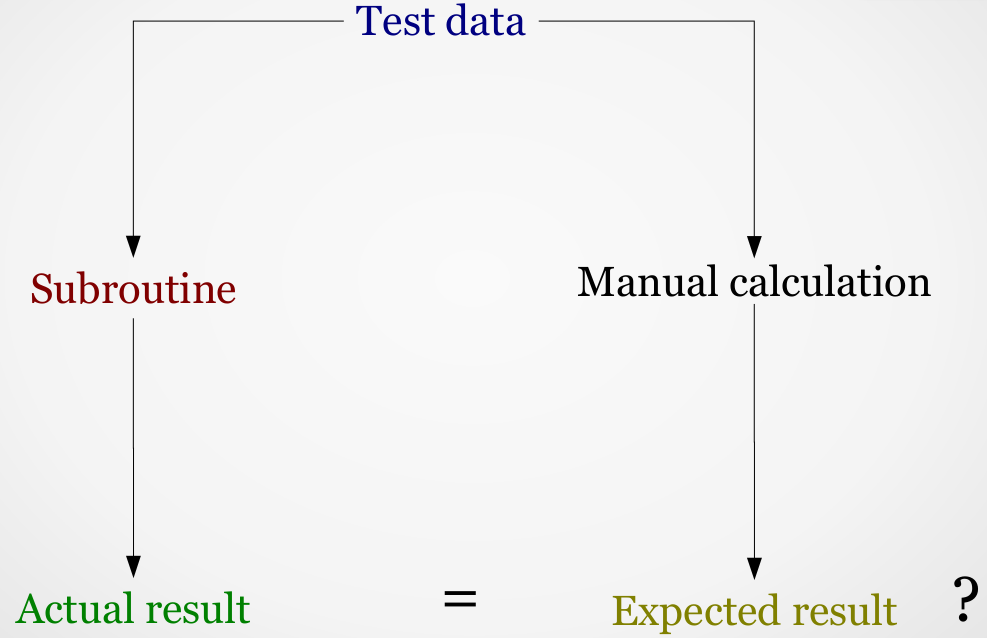# Introduction

#### Unit testing:

• A very important concept in all languages
• Like version control, it is often missing from introductory programming courses
• Here we focus on Python, but the concepts are general
• Testing and test-driven design

## What is Unit Testing?

#### "A method of testing individual 'units' of source code to determine whether they are fit for use"

• Unit testing requires modular design of your code
• Smaller subroutines/methods are easier to test
• More testing = fewer bugs!

## Why do it?

• Individual subroutines are easier to test than whole programs
• Unit tests can be standardized and automated
• Automated testing allows extensive testing after changes are made to code
• Tests also act as documentation for your code

## Anatomy of a test## A simple example

#### See example

```#!/usr/bin/env python3

# --------------------------------------------------------------------------
def signedSquare(val):
"""Returns the square of a number, but if the number was negative
the returned value is also negative
Input:  val  A number to be squared
Return:      the signed square of the number
"""

# Simple way of doing it - not very neat
if(val < 0):
sign = -1
else:
sign = 1

# Let's try the 'Conditional expressions' approach instead
# sign = -1 if val < 0 else 1

square = val * val

return(sign * square)

# --------------------------------------------------------------------------
# Test code

if __name__ == "__main__":

val = signedSquare(2)
if(val != 4):
print("signedSquare(2) failed")
else:
print("signedSquare(2) success")

val = signedSquare(0)
if(val != 0):
print("signedSquare(0) failed")
else:
print("signedSquare(0) success")

val = signedSquare(-2)
if(val != -4):
print("signedSquare(-2) failed")
else:
print("signedSquare(-2) success")

```

## Doing it better

#### See example

```#!/usr/bin/env python3

# --------------------------------------------------------------------------
def signedSquare(val):
"""Returns the square of a number, but if the number was negative
the returned value is also negative
Input:  val  A number to be squared
Return:      the signed square of the number
"""

sign = -1 if val < 0 else 1
square = val * val

return(sign * square)

# --------------------------------------------------------------------------
def runTestOneReturn(name, input, expected, actual):
"""Run a test on a subroutine that returns a single value.
Input is:
name     - the name of the subroutine being tested
input    - the input to that subroutine (in quotes if more than one item)
expected - the expected output from the subroutine
actual   - the actual output from the subroutine
"""

if(expected == actual):
print("Success: %s(%s)" % (name, input))
else:
print("Fail:    %s(%s) - expected %s, obtained %s" %
(name, input, expected, actual))

# --------------------------------------------------------------------------
def test_signedSquare():
"""Run tests on the signedSquare() routine"""
runTestOneReturn("signedSquare",  2,  4, signedSquare(2))
runTestOneReturn("signedSquare",  0,  0, signedSquare(0))
runTestOneReturn("signedSquare", -2, -4, signedSquare(-2))

# --------------------------------------------------------------------------
# MAIN PROGRAM: Run a set of tests
if __name__ == "__main__":
test_signedSquare()

```